Pure and Applied Mathematics Journal
Volume 4, Issue 4, August 2015, Pages: 155-158

Fixed Point Theorems for Occasionally Weakly Compatible Maps in Intuitionistic Fuzzy Semi- Metric Space

Harpreet Kaur1, Saurabh Manro2

1Department of Mathematics, Desh Bhagat University, Mandi Gobindgarh, India

2School of Mathematics and Computer Applications, Thapar University, Patiala, India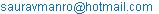(S. Manro)

Harpreet Kaur, Saurabh Manro. Fixed Point Theorems for Occasionally Weakly Compatible Maps in Intuitionistic Fuzzy Semi- Metric Space. Pure and Applied Mathematics Journal. Vol. 4, No. 4, 2015, pp. 155-158. doi: 10.11648/j.pamj.20150404.13

Abstract: In this paper, using the concept of occasionally weakly compatible maps, we prove common fixed point theorems for two maps and pairs of maps in intuitionistic fuzzy semi-metric space. Example is also given to prove the validity of proved results. Our results extends and generalizes various known fixed point theorems in the setting of metric, fuzzy, intuitionistic fuzzy and modified fuzzy metric spaces.

Keywords: Intuitionistic Fuzzy Semi- Metric Space, Occasionally Weakly Compatible Maps, Weakly Compatible Maps

Contents

1. Introduction

Atanassove introduced and studied the concept of intuitionistic fuzzy sets as a generalization of fuzzy sets. In 2004, Park defined the notion of intuitionistic fuzzy metric space with the help of continuous t-norms and continuous t-conorms. Recently, in 2006, Alaca et al. using the idea of Intuitionistic fuzzy sets, defined the notion of intuitionistic fuzzy metric space with the help of continuous t-norm and continuous t- conorms as a generalization of fuzzy metric space due to Kramosil and Michalek . In 2006, Turkoglu proved Jungck’s common fixed point theorem in the setting of intuitionistic fuzzy metric spaces for commuting mappings. Later, various authors (see, [7-12]) proved various fixed point theorems in the setting of intuitionistic fuzzy metric space. In this paper, using the concept of occasionally weakly compatible maps, we prove common fixed point theorems for two maps and pairs of maps in intuitionistic fuzzy semi-metric space. Example is also given to prove the validity of proved results. Our results extends and generalizes various known fixed point theorems (see, , ) in the setting of metric, fuzzy, intuitionistic fuzzy and modified fuzzy metric spaces.

2. Preliminaries

The concepts of triangular norms (t –norm) and triangular conorms (t- conorm) are known as the axiomatic skelton that we use are characterization fuzzy intersections and union respectively. These concepts were originally introduced by Menger in study of statistical metric spaces.

Definition 2.1: A binary operation * : [0,1]×[0,1] ® [0,1] is continuous t-norm if * is satisfies the following conditions: for all a, b, c, d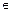[0,1],

(i) * is commutative and associative;

(ii) * is continuous;

(iii) a * 1 = a;

(iv) a * b £ c * d whenever a £ c and b £ d.

Definition 2.2: A binary operation ◊ : [0,1]×[0,1] ® [0,1] is continuous t-conorm if ◊ is satisfies the following conditions: for all a, b, c, d[0,1],

(i) ◊ is commutative and associative;

(ii) ◊ is continuous;

(iii) a ◊ 0 = a;

(iv) a ◊ b £ c ◊ d whenever a £ c and b £ d.

Alaca et al. defined the notion of intuitionistic fuzzy metric space as follows :

Definition 2.3: A 5-tuple (X, M, N, *, ◊) is said to be an intuitionistic fuzzy metric space if X is an arbitrary set, * is a continuous t-norm, ◊ is a continuous t-conorm and M, N are fuzzy sets on X2×[0, ∞) satisfying the following conditions: for all x, y, zX and s, t > 0,

(i) M(x, y, t) + N(x, y, t) £ 1;

(ii) M(x, y, 0) = 0;

(iii) M(x, y, t) = 1 if and only if x = y;

(iv) M(x, y, t) = M(y, x, t);

(v) M(x, y, t) * M(y, z, s) £ M(x, z, t + s);

(vi) M(x, y, .) : [0, ∞) → [0,1] is left continuous;

(vii) limt→∞M(x, y, t) = 1;

(viii) N(x, y, 0) = 1;

(ix) N(x, y, t) = 0 if and only if x = y;

(x) N(x, y, t) = N(y, x, t);

(xi) N(x, y, t) ◊ N(y, z, s) ≥ N(x, z, t + s);

(xii) N(x, y, .) : [0, ∞)→[0,1] is right continuous;

(xiii) limt→∞N(x, y, t) = 0.

Then (M, N) is called an intuitionistic fuzzy metric space on X. The functions M(x, y, t) and N(x, y, t) denote the degree of nearness and the degree of non-nearness between x and y w.r.t. t respectively.

If only (i), (ii), (iii), (iv), (viii), (ix), (x) holds, then the 5-tuple (X, M, N, *, ◊) is said to be fuzzy semi – metric (symmetric) space.

Remark 2.1. Every fuzzy metric space (X, M, *) is an intuitionistic fuzzy metric space of the form (X, M, 1-M, *, ◊) such that t-norm * and t-conorm ◊ are associated as                x ◊ y = 1-((1-x) * (1-y)) for all x, yX.

Lemma 2.1: In intuitionistic fuzzy metric space            (X, M, N, *, ◊),  M(x, y, .) is non-decreasing and N(x, y, .) is non-increasing for all x, yX.

Definition 2.4: Let (X, M, N, *, ◊) be an intuitionistic fuzzy semi-metric space. Then

(a) a sequence {xn} in X is said to be Cauchy sequence if, for all t > 0 and p > 0,

limn→∞M(xn+p, xn, t) = 1 and limn→∞N(xn+p, xn, t) = 0.

(b) a sequence {xn} in X is said to be convergent to a point xX if, for all t > 0,

limn→∞M(xn, x, t) = 1 and limn→∞N(xn, x, t) = 0.

Definition 2.5: An intuitionistic fuzzy semi-metric space (X, M, N, *, ◊) is said to be complete if and only if every Cauchy sequence in X is convergent.

Example 2.1: Let X = {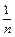: n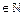}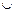{0} and let * be the continuous t-norm and ◊ be the continuous t-conorm defined by a * b = ab and a ◊ b = min{1, a + b} respectively, for all a, b in [0, 1]. For each t > 0 and x, yX, define         (M, N) by

M(x, y, t) =and

N(x, y, t) =Clearly, (X, M, N, *, ◊) is complete intuitionistic fuzzy metric space.

Definition 2.6: A pair of self mappings ( f , g ) of a intuitionistic fuzzy semi-metric space (X, M, N, *, ◊)  is said to be commuting if

M( fgx , gfx , t ) = 1 and

N( fgx , gfx , t ) = 0 for all x in X.

Definition 2.7: A pair of self mappings ( f , g ) of a intuitionistic fuzzy semi-metric space (X, M, N, *, ◊)  is said to be commuting if

M( fgx , gfx , t ) = 1 and N( fgx , gfx , t ) = 0 for all x in X.

Definition 2.8: A pair of self mappings ( f , g ) of a intuitionistic fuzzy semi-metric space (X, M, N, *, ◊) is said to be weakly commuting if

M( fgx , gfx , t ) ≥ M(fx, gx, t) and

N( fgx , gfx , t ) ≤ N(fx, gx, t)

for all x in X and t > 0.

Definition 2.9: A pair of self mappings (f, g ) of a intuitionistic fuzzy semi-metric space (X, M, N, *, ◊) is said to be compatible if

limn→∞M(fgxn, gfxn, t) = 1 and

limn→∞N(fgxn, gfxn, t) = 0 for all t > 0,

whenever {xn} is a sequence in X such that

limn→∞fxn = limn→∞ gxn = u for some u in X.

Definition 2.10: Let (X, M, N, *, ◊) be a intuitionistic fuzzy semi-metric space. f and g be self maps on X. A point x in X is called a coincidence point of f and g iff fx = gx. In this case, w = fx = gx is called a point of coincidence of f and g.

Definition 2.11: A pair of self mappings (f, g ) of a intuitionistic fuzzy semi-metric space (X, M, N, *, ◊) is said to be weakly compatible if they commute at the coincidence points i.e., if fu = gu for some u in X , then fgu = gfu.

It is easy to see that two compatible maps are weakly compatible but converse is not true.

Definition 2.12: Two self mappings f and g of a intuitionistic fuzzy semi-metric space (X, M, N, *, ◊) are said to be occasionally weakly compatible (owc) iff there is a point x in X which is coincidence point of f and g at which f and g commute.

Lemma 2.2: Let (X, M, N, *, ◊) be a intuitionistic fuzzy semi-metric space. f and g be owc self maps on X and let f and g have a unique point of coincidence, w = fx = gx, then w is the unique common fixed point of f and g.

3. Main Results

Let a function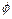be defined by: [0,1]→[0,1] satisfying the conditionfor all q < 1 and a function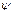be defined by: [0,1]→[0,1] satisfying the condition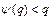for all q < 1.

Theorem 3.1: Let (X, M, N, *, ◊) be a intuitionistic fuzzy semi-metric space with continuous t-norm and continuous t- conorm defined by a * b = min { a, b } and a ◊ b = max {a, b} for all a, b in [0,1]. If f and g are owc self maps on X and

M(fx,fy,t) ≥[ min{ M(gx,gy,t), M(gx,fy,t), M(gy,fx,t), M(gy,fy,t) } ]

and

N(fx,fy,t) ≤[ max{ N(gx,gy,t), N(gx,fy,t), N(gy,fx,t),

N(gy,fy,t) } ]                                          (1)

for all x, y in X and t > 0. Then f and g have a unique common fixed point.

Proof: Since f and g are owc, there exist a point u in X such that fu = gu, fgu = gfu. We claim that fu is the unique common fixed point of f and g. We first assert that fu is a fixed point of f.

For, if ffu ≠ fu , then from equation (1), we get

M(fu,ffu,t) ≥[ min{ M(gu,gfu,t), M(gu,ffu,t), M(gfu,fu,t), M(gy,ffu,t) } ]

=[ min{ M(fu,ffu,t), M(fu,ffu,t), M(fu,ffu,t),       M(gfu,gfu,t) } ] =[ M(fu,ffu,t) ] > M(fu,ffu,t)

and

N(fu,ffu,t) ≤[ max{ N(gu,gfu,t), M(gu,ffu,t), N(gfu,fu,t), N(gy,ffu,t) } ]

=[ min{ N(fu,ffu,t), N(fu,ffu,t), N(fu,ffu,t),      N(gfu,gfu,t) } ] =[ N(fu,ffu,t) ] < N(fu,ffu,t)

this a contradiction. So ffu = fu and ffu = fgu = gfu = fu. Hence fu is a common fixed point of f and g.

Now we prove uniqueness. Suppose that u, v in X such that fu = gu = u and fv = gv = v and u ≠ v. Then from equation (1),

M(u,v,t) = M(fu,fv,t) ≥[ min{ M(gu,gv,t), M(gu,fv,t), M(gv,fu,t), M(gv,fv,t) } ] =[ min{ M(u,v,t), M(u,v,t), M(u,v,t), M(u,v, t) } ] =[ M(u,v,t) ] > M(u,v,t)

and

N(u,v,t) = N(fu,fv,t) ≤[ max{ N(gu,gv,t), N(gu,fv,t), N(gv,fu,t), N(gv,fv,t) } ]=[ max{ N(u,v,t), N(u,v,t), N(u,v,t), N(u,v, t) } ]=[ N(u,v,t) ] < N(u,v,t)

this is a contradiction. So u = v. Therefore, the common fixed point of f and g is unique.

Now, we give an example which satisfies the conditions of above theorem.

Example 3.1: Let X = [ 0, 1] and: X→X ,: X→X be defined as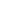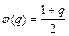and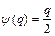, then(q) > q where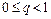,(q) < q where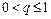, and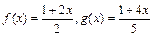. Clearly, f and g satisfies all the conditions w.r.t.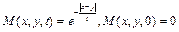and. In this example, f and g are owc maps and f and g satisfy the equation (1). f and g have a unique common fixed point x = 1.

Theorem 3.2: Let (X, M, N, *, ◊) be a intuitionistic fuzzy semi-metric space with continuous t-norm and continuous t- conorm defined by a * b = min { a, b } and a ◊ b = max {a, b} for all a, b in [0,1]. Suppose that f, g, S, T are self maps on X and the pairs {f , S} and {g , T} are owc. If

M(fx,gy,t) > min { M(Sx,Ty,t), M(Sx,fx,t), M(Ty,gy,t), M(Sx,gy,t), M(Ty,fx,t) } and

N(fx,gy,t) < max { N(Sx,Ty,t), N(Sx,fx,t), N(Ty,gy,t),                  N(Sx,gy,t), N(Ty,fx,t) },                           (2)

for all x, y in X and t > 0. Then f, g, S and T have a unique common fixed point.

Proof: By hypothesis, there exists points x, y in X such that fx = Sx and gy = Ty. Suppose that M(fx,gy,t) ≠ 1 and N(fx,gy,t) ≠ 0 for all t > 0. Then from equation (2)

M(fx,gy,t) > min { M(Sx,Ty,t), M(Sx,fx,t), M(Ty,gy,t), M(Sx,gy,t), M(Ty,fx,t) }= min { M(fx,gy,t), M(fx,fx,t), M(gy,gy,t), M(fx,gy,t), M(gy,fx,t) }= M(fx,gy,t)

N(fx,gy,t) < max { N(Sx,Ty,t), N(Sx,fx,t), N(Ty,gy,t), N(Sx,gy,t), N(Ty,fx,t) }= max { N(fx,gy,t), N(fx,fx,t), N(gy,gy,t), N(fx,gy,t), N(gy,fx,t) }= N(fx,gy,t)

this is a contradiction. Hence M(fx,gy,t) = 1 and N(fx,gy,t) = 0 for all t > 0. This implies that fx = gy. So fx = Sx = gy = Ty. Moreover, if there is another point z such that fz = Sz, then, using (2) it follows that fz = Sz = gy = Ty or fx = fz and w = fx = Sx is the unique point of coincidence of f and S. Then by Lemma 2.2, it follows that w is the unique common fixed point of f and S. By symmetry, there is a unique common fixed point z in X such that z = gz = Tz. Suppose that w ≠ z. Using (2),

M(w,z,t) = M(fw,gz,t) > min { M(Sw,Tz,t), M(Sw,fw,t), M(Tz,gz,t), M(Sw,gz,t), M(Tz,fw,t) }

M(w,z,t) > min { M(w,z,t), M(w,w,t), M(z,z,t), M(w,z,t), M(z,w,t) }

M(w,z,t) > M(w,z,t)

and

N(w,z,t) = N(fw,gz,t) < max { N(Sw,Tz,t), N(Sw,fw,t), N(Tz,gz,t), N(Sw,gz,t), N(Tz,fw,t) }

N(w,z,t) < max { N(w,z,t), N(w,w,t), N(z,z,t), N(w,z,t), N(z,w,t) }

N(w,z,t) < N(w,z,t)

This is a contradiction. Therefore w = z and w is a unique point of coincidence of f, g, S, T. By Lemma 2.2, w is the unique common fixed point of f, g, S, T.

4. Conclusion

In this paper, we proved two common fixed point theorems: one for two self maps and other for two pairs of self maps in the setting of intuitionistic fuzzy semi-metric space. Example is also given to prove the validity of main result. Proved results generalize and extend various known results (see, , ) in the literature.

References

1. C. Alaca, D. Turkoglu, and C. Yildiz, Fixed points in intuitionistic fuzzy metric spaces, Chaos, Solitons & Fractals, 29(2006), 1073-1078.
2. K. Atanassov, Intuitionistic fuzzy sets, Fuzzy Sets and System, 20(1986), 87-96.
3. H. Chandra and A. Bhatt, Fixed point theorems for occasionally weakly compatible maps in probabilistic semi-metric space, Int, Journal of Math. Analysis, 3(12)(2009), 563-570.
4. G. Jungck, Commuting mappings and fixed points, Amer. Math. Monthly, 83(1976), 261-263.
5. G. Jungck and B. E. Rhoades, Fixed point Theorems for occasionally weakly compatible mappings, Fixed point theory, 7(2006), 286-296.
6. I. Kramosil and J. Michalek, Fuzzy metric and statistical metric spaces, Kybernetica, 11(1975), 326-334.
7. S. Manro, S. Kumar and S. Singh, Common fixed point theorems in intuitionistic fuzzy metric spaces, Applied Mathematics,1(2010), 510-514.
8. S. Manro, S. S. Bhatia and S. Kumar, Common fixed point theorems for weakly compatible maps satisfying common (E.A.) property in intuitionistic fuzzy metric spaces using implicit relation, Journal of Advanced Studies in Topology, 3(2) (2012), 38-44.
9. S. Manro, H. Bouharjera and S. Singh, A common fixed point theorem in intuitionistic fuzzy metric space by using sub-compatible maps, Int. J. Contemp. Math. Sciences, 5(55) (2010) 2699 – 2707.
10. S. Manro, S. S. Bhatia and S. Kumar, Common fixed point theorem for weakly compatible maps satisfying E.A. property in intuitionistic fuzzy metric spaces, Punjab University Journal of Mathematics, 42(2010), 51-56.
11. S. Manro,Common fixed point theorem in intuitionistic fuzzy metric spaces using strict contractive condition, International Journal of Engineering and Technology, 2(1)(2012) 61-66.
12. S. Manro, S. Kumar and S.S. Bhatia, Common fixed point theorems in intuitionistic fuzzy metric spaces using occasionally weakly compatible maps, J. Math. Comput. Sci. , 2(2) (2012), 73-81.
13. J. H. Park, Intuitionistic fuzzy metric spaces, Chaos, Solitons & Fractals, 22(2004), 1039-1046.
14. D. Turkoglu, C. Alaca and C. Yildiz, Common fixed point theorems of compatible maps in intuitionistic fuzzy metric spaces, Southeast Asian Bulletin of Mathematics, 32(2008), 21-33.

 Contents 1. 2. 3. 4.
Article ToolsAbstractPDF(163K)